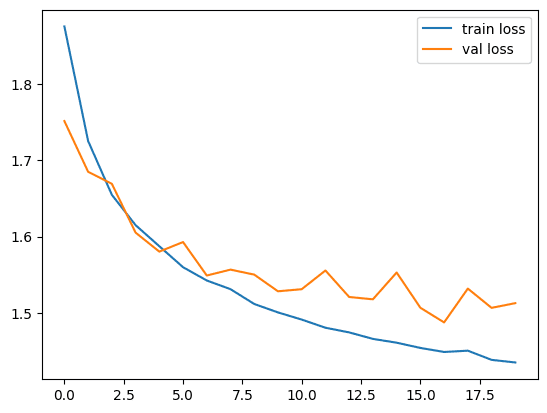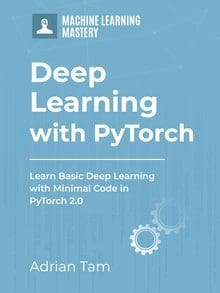SALE! Use code blackfriday for 40% off everything!
Hurry, sale ends soon! Click to see the full catalog.

# Building an Image Classifier with a Single-Layer Neural Network in PyTorch

A single-layer neural network, also known as a single-layer perceptron, is the simplest type of neural network. It consists of only one layer of neurons, which are connected to the input layer and the output layer. In case of an image classifier, the input layer would be an image and the output layer would be a class label.

To build an image classifier using a single-layer neural network in PyTorch, you’ll first need to prepare your data. This typically involves loading the images and labels into a PyTorch dataloader, and then splitting the data into training and validation sets. Once your data is prepared, you can define your neural network.

Next, you can use PyTorch’s built-in functions to train the network on your training data and evaluate its performance on your validation data. You’ll also need to pick an optimizer such as stochastic gradient descent (SGD) and a loss function like cross-entropy loss.

Note that a single layer neural network might not be ideal for every task, but it can be good as simple classifier and also can be helpful for you to understand the inner workings of the neural network and to be able to debug it.

So, let’s build our image classifier. In the process you’ll learn:

• How to use and preprocess built-in datasets in PyTorch.
• How to build and train custom neural networks in PyTorch.
• How to build a step-by-step image classifier in PyTorch.
• How to make predictions using the trained model in PyTorch.

Let’s get started.Building an Image Classifier with a Single-Layer Neural Network in PyTorch.
Picture by Alex Fung. Some rights reserved.

## Overview

This tutorial is in three parts; they are

• Preparing the Dataset
• Build the Model Architecture
• Train the Model

## Preparing the Dataset

In this tutorial, you will use the CIFAR-10 dataset. It is a dataset for image classification, consisting of 60,000 color images of 32×32 pixels in 10 classes, with 6,000 images per class. There are 50,000 training images and 10,000 test images. The classes include airplanes, cars, birds, cats, deer, dogs, frogs, horses, ships, and trucks. CIFAR-10 is a popular dataset for machine learning and computer vision research, as it is relatively small and simple, yet challenging enough to require the use of deep learning methods. This dataset can be easily imported into PyTorch library.

Here is how you do that.

You specified the root directory where the dataset should be downloaded, and setting train=True to import the training set, and train=False to import the test set. The download=True argument will download the dataset if it’s not already present in the specified root directory.

## Building the Neural Network Model

Let’s define a simple neural network SimpleNet that inherits from torch.nn.Module. The network has two fully connected (fc) layers, fc1 and fc2, defined in the __init__ method. The first fully connected layer fc1 takes in the image as input and has 100 hidden neurons. Similarly, the second fully connected layer fc2 has 100 input neurons and num_classes output neurons. The num_classes parameter defaults to 10 as there are 10 classes.

Moreover, the forward method defines the forward pass of the network, where the input x is passed through the layers defined in the __init__ method. The method first reshapes the input tensor x to have a desired shape using the view method. The input then passes through the fully connected layers along with their activation functions and, finally, an output tensor is returned.

Kick-start your project with my book Deep Learning with PyTorch. It provides self-study tutorials with working code.

Here is the code for all explained above.

And, write a function to visualize this data, which will also be useful when you train the model later.

Now, let’s instantiate the model object.

### Want to Get Started With Deep Learning with PyTorch?

Take my free email crash course now (with sample code).

Click to sign-up and also get a free PDF Ebook version of the course.

## Training the Model

You will create two instances of PyTorch’s DataLoader class, for training and testing respectively. In train_loader, you set the batch size at 64 and shuffle the training data randomly by setting shuffle=True.

Then, you will define the functions for cross entropy loss and Adam optimizer for training the model. You set the learning rate at 0.001 for the optimizer.

It is similar for test_loader, except we don’t need to shuffle.

Finally, let’s set a training loop to train our model for a few epochs. You will define some empty lists to store the values of the loss and accuracy metrices for loss and accuracy.

Running this loop will print you the following:

As you can see, the single-layer classifier is trained for only 20 epochs and it achieved a validation accuracy of around 47 percent. Train it for more epochs and you may get a decent accuracy. Similarly, our model had only a single layer with 100 hidden neurons. If you add some more layers, the accuracy may significantly improve.

Now, let’s plot loss and accuracy matrices to see how they look like.

The loss plot is like:And the accuracy plot is the following:Here is how you can see how the model make predictions against the true labels.

The printed labels are as following:

These labels are to correspond to the following images:## Summary

In this tutorial, you learned how you can build an image classifier using only a single-layer neural network. Particularly, you learned:

• How to use and preprocess built-in datasets in PyTorch.
• How to build and train custom neural networks in PyTorch.
• How to build a step-by-step image classifier in PyTorch.
• How to make predictions using the trained model in PyTorch.

## Get Started on Deep Learning with PyTorch!#### Learn how to build deep learning models

...using the newly released PyTorch 2.0 library

Discover how in my new Ebook:
Deep Learning with PyTorch

It provides self-study tutorials with hundreds of working code to turn you from a novice to expert. It equips you with
tensor operation, training, evaluation, hyperparameter optimization, and much more...

### 12 Responses to Building an Image Classifier with a Single-Layer Neural Network in PyTorch

1.Tony the Riger January 20, 2023 at 8:35 am #

2.Chuck February 2, 2023 at 8:08 am #

Model worked but the codes after this i.e.,
plottiungs for accuracy/loss and prediction image,
the kernal died with this message –
‘The kernel appears to have died. It will restart automatically’. I tried several times but the result were same. Any suggestion for solving this? Thanmks.

3.Leo February 24, 2023 at 8:23 am #

# Create the Data object
“dataset = Data()”

Pytorch reported Error after this. The Data Class was not created?

•James Carmichael February 24, 2023 at 11:03 am #

Hi Leo…Please elaborate on your question so that we may better assist you. That is…what errors are you receiving?

•Fabio April 20, 2023 at 8:16 pm #

in this tutorial it must have been missed, but it was present in other tutorials

use this before dataset = Data():

# Creating the dataset class
class Data(Dataset):
def __init__(self):
self.x = torch.arange(-2, 2, 0.1).view(-1, 1)
self.y = torch.zeros(self.x.shape, 1)
self.y[self.x[:, 0] > 0.2] = 1
self.len = self.x.shape

def __getitem__(self, idx):
return self.x[idx], self.y[idx]

def __len__(self):
return self.len

•James Carmichael April 21, 2023 at 9:29 am #

Thank you Fabio for your feedback and suggestion!

•Narae July 31, 2023 at 5:22 pm #

Hi,

I think the

dataset = Data()

part was left in the code by mistake? I doesn’t seemed to be used anywhere in the code below it, and the Data() method is never defined prior to its use.

•James Carmichael August 1, 2023 at 9:15 am #

Hi Narae…Thanks for the feedback!

4.Leo February 24, 2023 at 11:37 am #

Hi Kames,

The code and error are here:

————————————————-

import torch
import torchvision
import torchvision.transforms as transforms

# import the CIFAR-10 dataset

# Create the Data object
dataset = Data()

————————————————————–

NameError Traceback (most recent call last)
Input In , in ()
9 # Create the Data object
—> 10 dataset = Data()

NameError: name ‘Data’ is not defined

5.Sean O'Connor April 1, 2023 at 3:46 pm #

Even single neural network layers don’t scale well with width.
If the width is n then the number of multiply add operations required is n squared.
What starts off as something reasonable at say n=8 giving 64 multiply adds, starts to get unreasonable at n=256 giving 65536 multiply adds.
However by using a combining algorithm you can restrain the costs:
https://ai462qqq.blogspot.com/2023/03/switch-net-4-reducing-cost-of-neural.html

•James Carmichael April 2, 2023 at 6:21 am #

Thank you for your feedback and contribution Sean!